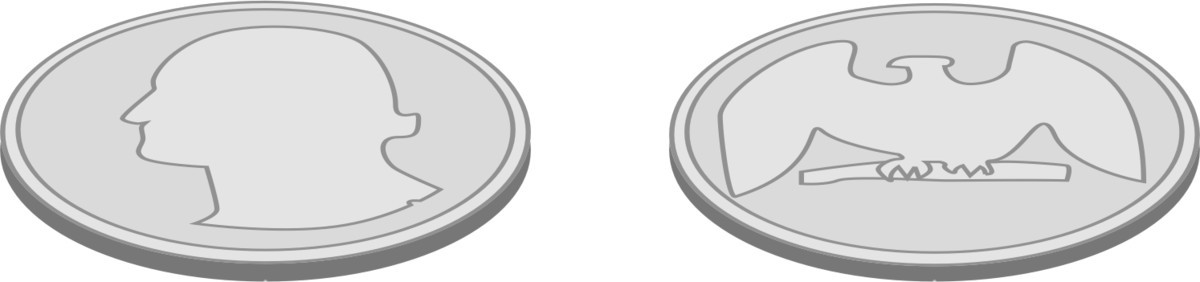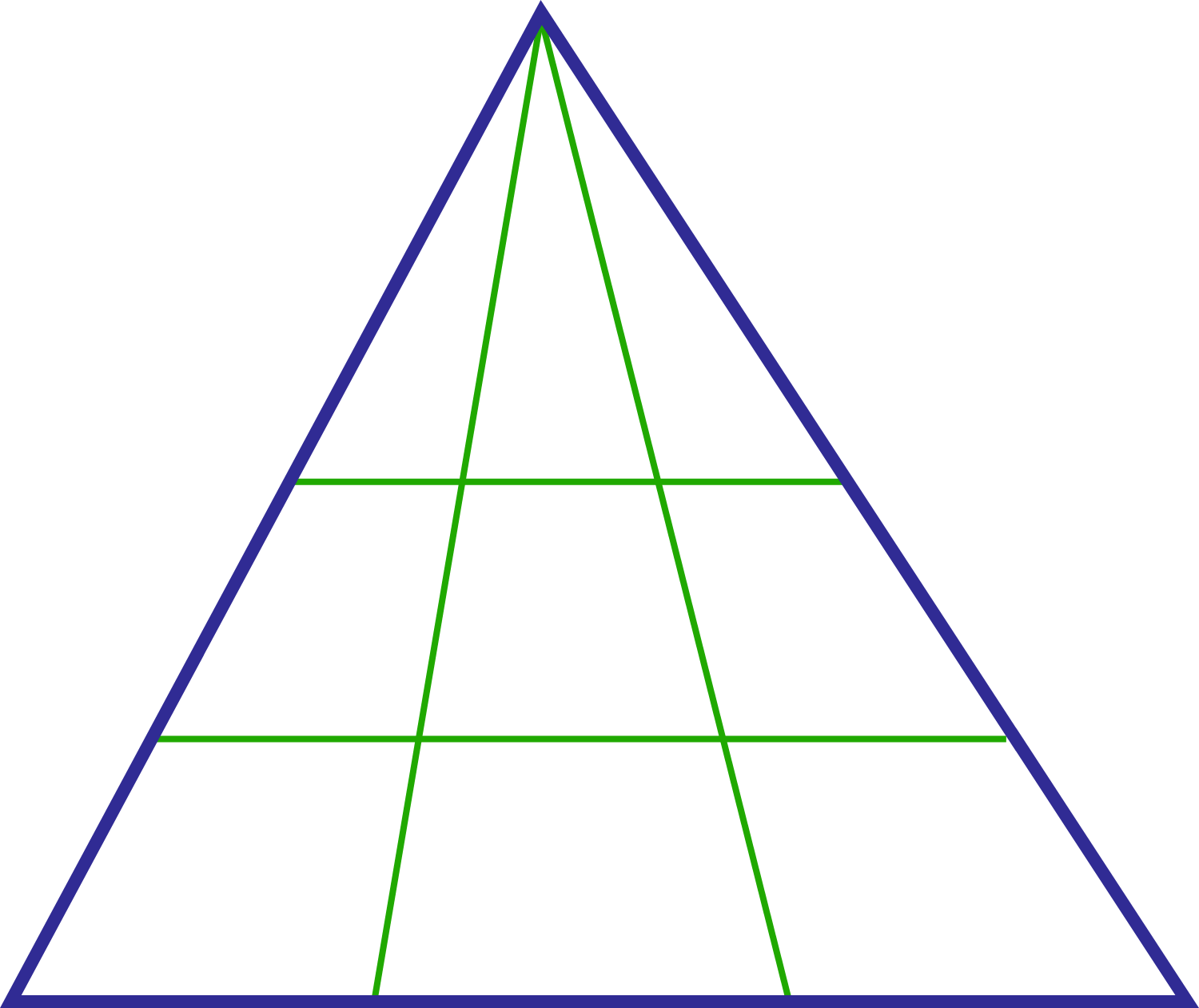Probability

# Discrete Mathematics Warmups: Level 2 ChallengesIf two fair coins are tossed simultaneously, what is the probability that exactly one head appears?

In a business meeting, a total of 45 handshakes occurred. How many people were at the meeting?

Assume that each person shakes hands with each other person exactly once.How many triangles are there in the above image?

A fair coin is tossed until it lands heads up. What is the probability that heads first appears on an odd numbered toss?If someone painted the outside of a $5 \times 5 \times 5$ cube made out of $1 \times 1 \times 1$ unit cubes, how many of the unit cubes would have paint on exactly 3 sides?

×

Problem Loading...

Note Loading...

Set Loading...